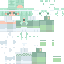### the lily girl ; te

Posted on: May 03, 2022
227
56
1
- + ❀ + - s h o w m o r e - + ❀ + -

Tell me what you think about the shading on the hair <3

.=*.=*.=*.=*.=*.=*.=*.=*.=*.=*.=*.=*.=*
- ❀ - Current followers 507 - ❀ -
Show Less

### the lily girl ; te

Posted on: May 03, 2022
227
56
1
- + ❀ + - s h o w m o r e - + ❀ + -

Tell me what you think about the shading on the hair <3

.=*.=*.=*.=*.=*.=*.=*.=*.=*.=*.=*.=*.=*
- ❀ - Current followers 507 - ❀ -